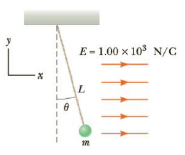Chapter 15, Problem 58AP

Chapter
Section
Textbook Problem

A small plastic ball of mass m = 2.00 g is suspended by a string of length L = 20.0 cm in a uniform electric field, as shown in Figure P15.52. If the ball is in equilibrium when the string makes a θ = 15.0° angle with the vertical as indicated, what is the net charge on the ball?Figure P15.58

To determine
The net charge on the ball.

Explanation

Given info: The mass of the ball is 2.0 g. The electric field strength is 1.00×103N/C .

The free body diagram is,

• Fe is the electric force.
• T is the tension.
• m is the mass of the ball.
• g is the acceleration due to gravity.

From the diagram,

Tcos15ο=mg

Tsin15ο=FE

Dividing the 2nd equation by the 1st,

FE=mgtan15ο

Formula to calculate the electric force is,

FE=qE

• E is the electric field.
• q is the charge on the ball.

Therefore,

qE=mgtan15ο

On Re-arranging,

q=mgtan15οE

Substitute 9.8ms2 for g, 1

Still sussing out bartleby?

Check out a sample textbook solution.

See a sample solution

The Solution to Your Study Problems

Bartleby provides explanations to thousands of textbook problems written by our experts, many with advanced degrees!

Get Started